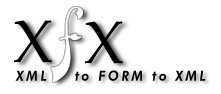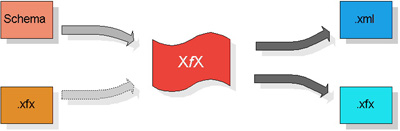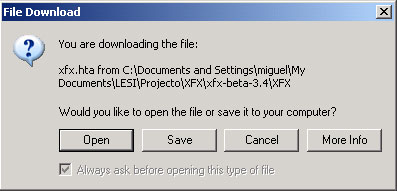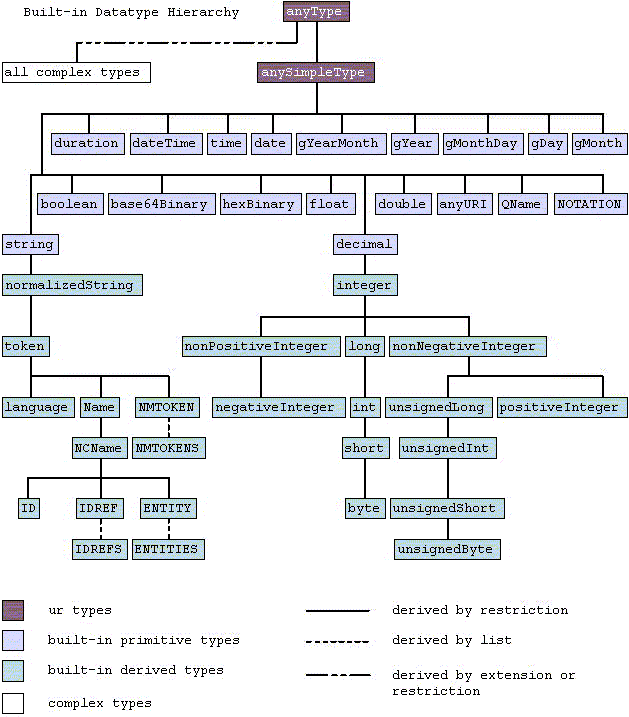University of Minho
Miguel Ferreira, Rui Guerra, Pedro Ferreira.

1 What is XfX?
2 Requirements
3 Features
4 Limitations
5 Getting Started
6 Feedback
7 Datatypes
7.1 Primitive datatypes
7.1.1 string
7.1.2 boolean
7.1.3 decimal
7.1.4 float
7.1.5 double
7.1.6 duration
7.1.7 dateTime
7.1.8 time
7.1.9 date
7.1.10 gYearMonth
7.1.11 gYear
7.1.12 gMonthDay
7.1.13 gDay
7.1.14 gMonth
7.1.15 hexBinary
7.1.16 base64Binary
7.1.17 anyURI
7.1.18 QName
7.1.19 NOTATION
7.2 Derived datatypes
7.2.1 normalizedString
7.2.2 token
7.2.3 language
7.2.4 NMTOKEN
7.2.5 NMTOKENS
7.2.6 Name
7.2.7 NCName
7.2.8 ID
7.2.9 IDREF
7.2.10 IDREFS
7.2.11 ENTITY
7.2.12 ENTITIES
7.2.13 integer
7.2.14 nonPositiveInteger
7.2.15 negativeInteger
7.2.16 long
7.2.17 int
7.2.18 short
7.2.19 byte
7.2.20 nonNegativeInteger
7.2.21 unsignedLong
7.2.22 unsignedInt
7.2.23 unsignedShort
7.2.24 unsignedByte
7.2.25 positiveInteger
7.3 Datatype examples

1 What is XfX?[toc]

XfX is a powerful editor for creating and editing XML documents based on the definition of the document, that is, the schema. For each document is generated an HTML form to enter or edit the data. In the form, boxes are painted with distinct color to differentiate each data structure.

The result is a XML document well-formed and validated, compliant with the schema of the XML document.fig. 1. XfX block diagram

2 Requirements[toc]

To write a XML document in XfX, you need the following:

• The XfX apllication itself
• A Web browser that supports Javascript and XML technologies:
• Stylesheet processor
• XML parser

This product was developed and fully tested with Microsoft© Internet Explorer© 6.0. It is possible that this application also runs on other browsers and/or operating systems rather than Microsoft's, althought we have not tested it. Any reports sent on this concern will be apretiated.

3 Features[toc]

• Datatype validation
• Support W3C Schemas;
• Portability;
• Validate and test well-formed XML documents according to the respective Schema;
• Generate a XML document;

4 Limitations[toc]

• Does not support recursive schema definitions;
• Does not validate Datatype restrictions;
• Does not support SimpleContent and ComplexContent definitions;
• Does not support Group elements ???

5 Getting Started[toc]

There are no instalation requirements what so ever. Just uncompress the package holding the XfX files into a directory and you're ready to go.

XfX uses Javascript to dynamicaly change the HTML form contents and perform datatype validation. Because of that, it works nicely on any workstation. It runs completly on the client-side and does not need any special HTTP server.

When running XfX on IE6 the following message appears just before entering XfX. This happens because XfX needs your permition to lower your browser's security. XfX needs to do that because it needs to write your disk so that you can save your documents.

Here is a screenshot of the generated message. Note that it isn't very explicit but that isn´t our faultfig. 2. HTA message.

If you don't trust our application just chose Cancel. Either way, you can analyse and trace all the XfX source code before you trust it enought to use.

6 Feedback[toc]

Please send any bug report, suggestion, question or comment to the authors at the following addresses:

7 Datatypes[toc]

The following image and datatype definitions were extracted form the W3C Recommendation on 02 May 2001, XML Schema Part 2: Datatypes.fig. 3. Datatype hierarchy (www.w3c.org).

7.1.1 string [toc]

The string datatype represents character strings in XML. The ·value space· of string is the set of finite-length sequences of characters (as defined in [XML 1.0 (Second Edition)]) that ·match· the Char production from [XML 1.0 (Second Edition)]. A character is an atomic unit of communication; it is not further specified except to note that every character has a corresponding Universal Character Set code point, which is an integer. see example

7.1.2 boolean [toc]

boolean has the ·value space· required to support the mathematical concept of binary-valued logic: {true, false}. see example

7.1.3 decimal [toc]

decimal represents arbitrary precision decimal numbers. The value space of decimal is the set of the values i × 10^-n, where i and n are integers such that n >= 0. The order-relation on decimal is: x < y iff y - x is positive.

The value space of types derived from decimal with a value for ·totalDigits· of p is the set of values i × 10^-n, where n and i are integers such that p >= n >= 0 and the number of significant decimal digits in i is less than or equal to p.

The ·value space· of types derived from decimal with a value for fractionDigits of s is the set of values i × 10^-n, where i and n are integers such that 0 <= n <= s. see example

7.1.4 float [toc]

float corresponds to the IEEE single-precision 32-bit floating point type [IEEE 754-1985]. The basic ·value space· of float consists of the values m × 2^e, where m is an integer whose absolute value is less than 2^24, and e is an integer between -149 and 104, inclusive. In addition to the basic ·value space· described above, the ·value space· of float also contains the following special values: positive and negative zero, positive and negative infinity and not-a-number. The ·order-relation· on float is: x < y iff y - x is positive. Positive zero is greater than negative zero. Not-a-number equals itself and is greater than all float values including positive infinity. see example

7.1.5 double [toc]

The double datatype corresponds to IEEE double-precision 64-bit floating point type [IEEE 754-1985]. The basic ·value space· of double consists of the values m × 2^e, where m is an integer whose absolute value is less than 2^53, and e is an integer between -1075 and 970, inclusive. In addition to the basic ·value space· described above, the ·value space· of double also contains the following special values: positive and negative zero, positive and negative infinity and not-a-number. The ·order-relation· on double is: x < y iff y - x is positive. Positive zero is greater than negative zero. Not-a-number equals itself and is greater than all double values including positive infinity. see example

7.1.6 duration [toc]

duration represents a duration of time. The ·value space· of duration is a six-dimensional space where the coordinates designate the Gregorian year, month, day, hour, minute, and second components defined in § 5.5.3.2 of [ISO 8601], respectively. These components are ordered in their significance by their order of appearance i.e. as year, month, day, hour, minute, and second. see example

7.1.7 dateTime [toc]

dateTime represents a specific instant of time. The ·value space· of dateTime is the space of Combinations of date and time of day values as defined in § 5.4 of [ISO 8601]. see example

7.1.8 time [toc]

time represents an instant of time that recurs every day. The ·value space· of time is the space of time of day values as defined in § 5.3 of [ISO 8601]. Specifically, it is a set of zero-duration daily time instances. see example

7.1.9 date [toc]

date represents a calendar date. The ·value space· of date is the set of Gregorian calendar dates as defined in § 5.2.1 of [ISO 8601]. Specifically, it is a set of one-day long, non-periodic instances e.g. lexical 1999-10-26 to represent the calendar date 1999-10-26, independent of how many hours this day has. see example

7.1.10 YearMonth [toc]

gYearMonth represents a specific gregorian month in a specific gregorian year. The ·value space· of gYearMonth is the set of Gregorian calendar months as defined in § 5.2.1 of [ISO 8601]. Specifically, it is a set of one-month long, non-periodic instances e.g. 1999-10 to represent the whole month of 1999-10, independent of how many days this month has. see example

7.1.11 gYear [toc]

gYear represents a gregorian calendar year. The ·value space· of gYear is the set of Gregorian calendar years as defined in § 5.2.1 of [ISO 8601]. Specifically, it is a set of one-year long, non-periodic instances e.g. lexical 1999 to represent the whole year 1999, independent of how many months and days this year has. see example

7.1.12 gMonthDay [toc]

gMonthDay is a gregorian date that recurs, specifically a day of the year such as the third of May. Arbitrary recurring dates are not supported by this datatype. The ·value space· of gMonthDay is the set of calendar dates, as defined in § 3 of [ISO 8601]. Specifically, it is a set of one-day long, annually periodic instances. see example

7.1.13 gDay [toc]

gDay is a gregorian day that recurs, specifically a day of the month such as the 5th of the month. Arbitrary recurring days are not supported by this datatype. The ·value space· of gDay is the space of a set of calendar dates as defined in § 3 of [ISO 8601]. Specifically, it is a set of one-day long, monthly periodic instances. see example

7.1.14 gMonth [toc]

gMonth is a gregorian month that recurs every year. The ·value space· of gMonth is the space of a set of calendar months as defined in § 3 of [ISO 8601]. Specifically, it is a set of one-month long, yearly periodic instances. see example

7.1.15 hexBinary [toc]

hexBinary represents arbitrary hex-encoded binary data. The ·value space· of hexBinary is the set of finite-length sequences of binary octets. see example

7.1.16 base64Binary [toc]

base64Binary represents Base64-encoded arbitrary binary data. The ·value space· of base64Binary is the set of finite-length sequences of binary octets. For base64Binary data the entire binary stream is encoded using the Base64 Content-Transfer-Encoding defined in Section 6.8 of [RFC 2045]. see example

7.1.17 anyURI [toc]

anyURI represents a Uniform Resource Identifier Reference (URI). An anyURI value can be absolute or relative, and may have an optional fragment identifier (i.e., it may be a URI Reference). This type should be used to specify the intention that the value fulfills the role of a URI as defined by [RFC 2396], as amended by [RFC 2732]. see example

7.1.18 QName [toc]

QName represents XML qualified names. The ·value space· of QName is the set of tuples {namespace name, local part}, where namespace name is an anyURI and local part is an NCName. The ·lexical space· of QName is the set of strings that ·match· the QName production of [Namespaces in XML]. see example

7.1.19 NOTATION [toc]

NOTATION represents the NOTATION attribute type from [XML 1.0 (Second Edition)]. The ·value space· of NOTATION is the set QNames. The ·lexical space· of NOTATION is the set of all names of notations declared in the current schema. see example

7.2.1 normalizedString [toc]

normalizedString represents white space normalized strings. The ·value space· of normalizedString is the set of strings that do not contain the carriage return (#xD), line feed (#xA) nor tab (#x9) characters. The ·lexical space· of normalizedString is the set of strings that do not contain the carriage return (#xD) nor tab (#x9) characters. The ·base type· of normalizedString is string. see example

7.2.2 token [toc]

token represents tokenized strings. The ·value space· of token is the set of strings that do not contain the line feed (#xA) nor tab (#x9) characters, that have no leading or trailing spaces (#x20) and that have no internal sequences of two or more spaces. The ·lexical space· of token is the set of strings that do not contain the line feed (#xA) nor tab (#x9) characters, that have no leading or trailing spaces (#x20) and that have no internal sequences of two or more spaces. The ·base type· of token is normalizedString. see example

7.2.3 language [toc]

language represents natural language identifiers as defined by [RFC 1766]. The ·value space· of language is the set of all strings that are valid language identifiers as defined in the language identification section of [XML 1.0 (Second Edition)]. The ·lexical space· of language is the set of all strings that are valid language identifiers as defined in the language identification section of [XML 1.0 (Second Edition)]. The ·base type· of language is token. see example

7.2.4 NMTOKEN [toc]

NMTOKEN represents the NMTOKEN attribute type from [XML 1.0 (Second Edition)]. The ·value space· of NMTOKEN is the set of tokens that ·match· the Nmtoken production in [XML 1.0 (Second Edition)]. The ·lexical space· of NMTOKEN is the set of strings that ·match· the Nmtoken production in [XML 1.0 (Second Edition)]. The ·base type· of NMTOKEN is token. see example

7.2.5 NMTOKENS [toc]

NMTOKENS represents the NMTOKENS attribute type from [XML 1.0 (Second Edition)]. The ·value space· of NMTOKENS is the set of finite, non-zero-length sequences of ·NMTOKEN·s. The ·lexical space· of NMTOKENS is the set of white space separated lists of tokens, of which each token is in the ·lexical space· of NMTOKEN. The ·itemType· of NMTOKENS is NMTOKEN. see example

7.2.6 Name [toc]

Name represents XML Names. The ·value space· of Name is the set of all strings which ·match· the Name production of [XML 1.0 (Second Edition)]. The ·lexical space· of Name is the set of all strings which ·match· the Name production of [XML 1.0 (Second Edition)]. The ·base type· of Name is token. see example

7.2.7 NCName [toc]

NCName represents XML "non-colonized" Names. The ·value space· of NCName is the set of all strings which ·match· the NCName production of [Namespaces in XML]. The ·lexical space· of NCName is the set of all strings which ·match· the NCName production of [Namespaces in XML]. The ·base type· of NCName is Name. see example

7.2.8 ID [toc]

ID represents the ID attribute type from [XML 1.0 (Second Edition)]. The ·value space· of ID is the set of all strings that ·match· the NCName production in [Namespaces in XML]. The ·lexical space· of ID is the set of all strings that ·match· the NCName production in [Namespaces in XML]. The ·base type· of ID is NCName. see example

7.2.9 IDREF [toc]

IDREF represents the IDREF attribute type from [XML 1.0 (Second Edition)]. The ·value space· of IDREF is the set of all strings that ·match· the NCName production in [Namespaces in XML]. The ·lexical space· of IDREF is the set of strings that ·match· the NCName production in [Namespaces in XML]. The ·base type· of IDREF is NCName. see example

7.2.10 IDREFS [toc]

IDREFS represents the IDREFS attribute type from [XML 1.0 (Second Edition)]. The ·value space· of IDREFS is the set of finite, non-zero-length sequences of IDREFs. The ·lexical space· of IDREFS is the set of white space separated lists of tokens, of which each token is in the ·lexical space· of IDREF. The ·itemType· of IDREFS is IDREF. see example

7.2.11 ENTITY [toc]

ENTITY represents the ENTITY attribute type from [XML 1.0 (Second Edition)]. The ·value space· of ENTITY is the set of all strings that ·match· the NCName production in [Namespaces in XML] and have been declared as an unparsed entity in a document type definition. The ·lexical space· of ENTITY is the set of all strings that ·match· the NCName production in [Namespaces in XML]. The ·base type· of ENTITY is NCName. see example

7.2.12 ENTITIES [toc]

ENTITIES represents the ENTITIES attribute type from [XML 1.0 (Second Edition)]. The ·value space· of ENTITIES is the set of finite, non-zero-length sequences of ·ENTITY·s that have been declared as unparsed entities in a document type definition. The ·lexical space· of ENTITIES is the set of white space separated lists of tokens, of which each token is in the ·lexical space· of ENTITY. The ·itemType· of ENTITIES is ENTITY. see example

7.2.13 integer [toc]

integer is ·derived· from decimal by fixing the value of ·fractionDigits· to be 0. This results in the standard mathematical concept of the integer numbers. The ·value space· of integer is the infinite set {...,-2,-1,0,1,2,...}. The ·base type· of integer is decimal. see example

7.2.14 nonPositiveInteger [toc]

nonPositiveInteger is ·derived· from integer by setting the value of ·maxInclusive· to be 0. This results in the standard mathematical concept of the non-positive integers. The ·value space· of nonPositiveInteger is the infinite set {...,-2,-1,0}. The ·base type· of nonPositiveInteger is integer. see example

7.2.15 negativeInteger [toc]

negativeInteger is ·derived· from nonPositiveInteger by setting the value of ·maxInclusive· to be -1. This results in the standard mathematical concept of the negative integers. The ·value space· of negativeInteger is the infinite set {...,-2,-1}. The ·base type· of negativeInteger is nonPositiveInteger. see example

7.2.16 long [toc]

long is ·derived· from integer by setting the value of ·maxInclusive· to be 9223372036854775807 and ·minInclusive· to be -9223372036854775808. The ·base type· of long is integer. see example

7.2.17 int [toc]

int is ·derived· from long by setting the value of ·maxInclusive· to be 2147483647 and ·minInclusive· to be -2147483648. The ·base type· of int is long. see example

7.2.18 short [toc]

short is ·derived· from int by setting the value of ·maxInclusive· to be 32767 and ·minInclusive· to be -32768. The ·base type· of short is int. see example

7.2.19 byte [toc]

byte is ·derived· from short by setting the value of ·maxInclusive· to be 127 and ·minInclusive· to be -128. The ·base type· of byte is short. see example

7.2.20 nonNegativeInteger [toc]

nonNegativeInteger is ·derived· from integer by setting the value of ·minInclusive· to be 0. This results in the standard mathematical concept of the non-negative integers. The ·value space· of nonNegativeInteger is the infinite set {0,1,2,...}. The ·base type· of nonNegativeInteger is integer. see example

7.2.21 unsignedLong [toc]

unsignedLong is ·derived· from nonNegativeInteger by setting the value of ·maxInclusive· to be 18446744073709551615. The ·base type· of unsignedLong is nonNegativeInteger. see example

7.2.22 unsignedInt [toc]

unsignedInt is ·derived· from unsignedLong by setting the value of ·maxInclusive· to be 4294967295. The ·base type· of unsignedInt is unsignedLong. see example

7.2.23 unsignedShort [toc]

unsignedShort is ·derived· from unsignedInt by setting the value of ·maxInclusive· to be 65535. The ·base type· of unsignedShort is unsignedInt. see example

7.2.24 unsignedByte [toc]

unsignedByte is ·derived· from unsignedShort by setting the value of ·maxInclusive· to be 255. The ·base type· of unsignedByte is unsignedShort. see example

7.2.25 positiveInteger [toc]

positiveInteger is ·derived· from nonNegativeInteger by setting the value of ·minInclusive· to be 1. This results in the standard mathematical concept of the positive integer numbers. The ·value space· of positiveInteger is the infinite set {1,2,...}. The ·base type· of positiveInteger is nonNegativeInteger. see example

7.3 Datatype examples[toc]

Simple Type Examples (delimited by commas)
string Confirm this is electric
normalizedString Confirm this is electric
token Confirm this is electric
byte -1, 126
unsignedByte 0, 126
base64Binary GpM7
hexBinary 0FB7
integer -126789, -1, 0, 1, 126789
positiveInteger 1, 126789
negativeInteger -126789, -1
nonNegativeInteger 0, 1, 126789
nonPositiveInteger -126789, -1, 0
int -1, 126789675
unsignedInt 0, 1267896754
long -1, 12678967543233
unsignedLong 0, 12678967543233
short -1, 12678
unsignedShort 0, 12678
decimal -1.23, 0, 123.4, 1000.00
float -INF, -1E4, -0, 0, 12.78E-2, 12, INF, NaN
double -INF, -1E4, -0, 0, 12.78E-2, 12, INF, NaN
boolean true, false
1, 0
time 13:20:00.000, 13:20:00.000-05:00
dateTime 1999-05-31T13:20:00.000-05:00
duration P1Y2M3DT10H30M12.3S
date 1999-05-31
gMonth --05--
gYear 1999
gYearMonth 1999-02
gDay ---31
gMonthDay --05-31
Name shipTo
anyURI http://www.example.com/, http://www.example.com/doc.html#ID5
language en-GB, en-US, fr
ID
IDREF
IDREFS
ENTITY
ENTITIES
NOTATION
NMTOKEN US,
Brésil
NMTOKENS US UK,